# JSP Expression Language (EL) Tutorial

## What is Expression Language (EL)?

Expression Language (EL) is mechanism that simplifies the accessibility of the data stored in Java bean component and other object like request, session and application, etc. There are many operators in JSP that are used in EL like arithmetic and logical operators to perform an expression. It was introduced in JSP 2.0

In this tutorial, you will learn-

## JSP Syntax of Expression Language (EL)

Syntax of EL :\$(expression)

• In JSP, whatever present in the braces gets evaluated at runtime sent to the output stream.
• The expression is a valid EL expression and it can be mixed with a static text and can be combined with other expression to form larger expression.

To get a better idea, on how expression works in JSP, we will see below example.

In this example, we will see how EL is used as an operator to add two numbers (1+2) and get the output respectively.

```<%@ page language="java" contentType="text/html; charset=ISO-8859-1"
pageEncoding="ISO-8859-1"%>
<!DOCTYPE html PUBLIC "-//W3C//DTD HTML 4.01 Transitional//EN" "http://www.w3.org/TR/html4/loose.dtd">
<html>
<meta http-equiv="Content-Type" content="text/html; charset=ISO-8859-1">
<title>Guru JSP1</title>
<body>
<a>Expression is:</a>
\${1+2};
</body>
</html>```

Explanation of Code:

• Code Line 11: Expression Language (EL) is set where we are adding two numbers 1+2, hence it will give output as 3.

When you execute the above code, you will have the following Output.

Output: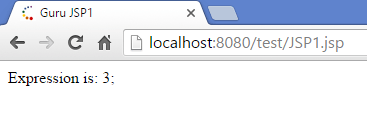• Expression is: 3 (As numbers 1+2 will be added and serve as an output)

Flow Control Statements:

JSP provides the power of Java to be embedded in the application. We can use all the APIs and building blocks of Java in JSP programming including control flow statements which include decision making and the loop statements.

There are two types of flow control statements described below;

1. Decision-Making statements
2. Loop Statements

Decision-Making Statements:

Decision-making statement in JSP is based on whether the condition set is true or false. The statement will behave accordingly.

There are two types of decision-making statements described below:

• If – else
• switch
• JSP If-else
• “If else” statement is basic of all control flow statements, and it tells the program to execute the certain section of code only if the particular test evaluates to true.
• This condition is used to test for more than one condition whether they are true or false.
• If the first condition is true then “if block” is executed and
• if it is false then “else block” is executed
• Syntax for if – else statement:
• If(test condition) { //Block of statements } else { //Block of statements }
• In this example,
• We are going to test “if else” condition by taking variable and checking the value if the variable matches with what it is initialized:
• <%@ page language=“java” contentType=“text/html; charset=ISO-8859-1”
• pageEncoding=“ISO-8859-1”%>
• <!DOCTYPE html PUBLIC “-//W3C//DTD HTML 4.01 Transitional//EN” “http://www.w3.org/TR/html4/loose.dtd”>
• <html>
•
•
• <meta http-equiv=“Content-Type” content=“text/html; charset=ISO-8859-1”>
• <title>Guru JSP2</title>
•
•
•
• <body>
•
• <%! int month=5; %>
•
• <% if(month==2){ %>
•
• <a>Its February</a>
•
• <% }else{ %>
•
• <a>Any month other than February</a>
•
• <%} %>
•
• </body>
•
•
• </html>
• Explanation of the code:
• Code Line 10: Variable named month is initialized to 5 in the expression tags
• Code Line 11: In the EL tags there is “if condition” stating that if the month is equal 2 (condition is tested here as true or false)
• Code Line 12: If the condition is true that is the variable month is 2 then it will print to output stream
• Code Line 13-15: If the above if condition fails then it will move to else part for all other cases where the statement will print to the output stream and condition is closed
• When you execute the above code, you will have the following Output.
• Output:
•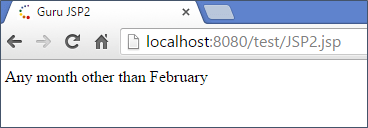• Since the month we have is 5 which is not equal to #2 (February). Hence, we have the output “Any month other than February” (month is mentioned as 5 hence else are executed)
• JSP Switch
• The body of the switch statement is called as a “switch block”.
• The switch case is used to check the number of possible execution paths.
• A switch can be used with all data types
• The switch statements contain more than one cases and one default case
• It evaluates the expression then executes all the statements following the matching case
• Syntax for switch statement:
• switch (operator) { Case 1: Block of statements break; Case 2: Block of statements break; case n: Block of statements break; default: Block of statements break; }
• Switch block begins with one parameter, which is the operator that needs to be passed and
• Then there are different cases which provides condition and whichever matches with the operator that case is executed.
• In below example,
• We have defined a variable week, and it is matched with the case whichever is true.In this case, week is 2 hence 2nd case is matched, and the output is Tuesday:
• <%@ page language=“java” contentType=“text/html; charset=ISO-8859-1”
• pageEncoding=“ISO-8859-1”%>
• <!DOCTYPE html PUBLIC “-//W3C//DTD HTML 4.01 Transitional//EN” “http://www.w3.org/TR/html4/loose.dtd”>
•
• <html>
•
•
• <meta http-equiv=“Content-Type” content=“text/html; charset=ISO-8859-1”>
•
• <title>Guru JSP3</title>
•
•
• <body>
•
• <%! int week=2; %>
•
• <% switch(week){
•
• case 0:
•
• out.println(“Sunday”);
•
• break;
•
• case 1:
•
• out.println(“Monday”);
•
• break;
•
• case 2:
•
• out.println(“Tuesday”);
•
• break;
•
• case 3:
•
• out.println(“wednesday”);
•
• break;
•
• case 4:
•
• out.println(“Thursday”);
•
• break;
•
• case 5:
•
• out.println(“Friday”);
•
• break;
•
• default:
•
• out.println(“Saturday”);
•
• }
•
• %>
•
• </body>
•
• </html>
• Explanation of Code:
• Code Line 10: Variable named week is initialized to 2 in the expression tags
• Code Line 11: In the EL tags the switch case is started where week is passed as parameter
• Code Line 12 – 29: All the cases have been mentioned starting from case 0 to case 5 where the value of week parameter is matched with the cases, and accordingly the output is print. In this case, the value is 2 hence case 2 will be executed in this case. Here “out” is the class of JSP which writes the output stream for generated response and “println” is a method of that class.
• Code Line 30-32: If the above all cases fails then it will move to default part and will get executed, where the statement will print to the output stream and condition is closed
• When you execute the above code, you will have the following Output.
• Output:
•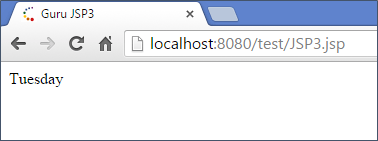• The output in this case is Tuesday as 2nd case is called.
• Loop Statements
• JSP For loop
• It is used for iterating the elements for a certain condition, and it has three parameters.
• Variable counter is initialized
• Condition till the loop has to be executed
• Counter has to be incremented
• For loop Syntax:
• For(inti=0;i<n;i++) { //block of statements }
• In this Example,
• We have for loop which iterates till counter is less than the given number:
• <%@ page language=“java” contentType=“text/html; charset=ISO-8859-1”
• pageEncoding=“ISO-8859-1”%>
• <!DOCTYPE html PUBLIC “-//W3C//DTD HTML 4.01 Transitional//EN” “http://www.w3.org/TR/html4/loose.dtd”>
• <html>
•
•
• <meta http-equiv=“Content-Type” content=“text/html; charset=ISO-8859-1”>
•
• <title>Guru JSP4</title>
•
•
• <body>
•
• <%! int num=5; %>
•
• <% out.println(“Numbers are:”);
•
• for(int i=0;i<num;i++){
•
• out.println(i);
•
• }%>
•
• </body>
•
• </html>
• Explanation for the code:
• Code Line 10: Variable named “num” is initialized to 5 in the expression tags
• Code Line 11-14: In the EL tags, “out” is the class of JSP and “println” is the method of out which prints in output stream and for loop is started which has three parameters:
• Variable i is initialized to 0,
• Condition is given where i to be less than local variable num,
• And i is incremented every time loop iterates.
• In the body of “forloop”, there is out a class of JSP which prints into the output stream using method println where we are printing the variable i.
• When you execute the above code, you will have the following Output.
• Output:
•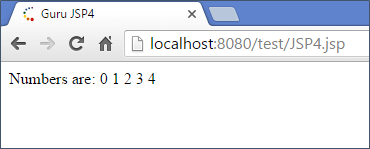• Output Numbers are 0 1 2 3 4. In this example, we are giving the condition that till the counter is less than equal to a variable the “for loop” has to be executed. The num is 5 so the loop will start from 0 and run till 4 (5 times). Hence the output.
• JSP While loop
• It is used to iterate the elements wherein it has one parameter of the condition.
• Syntax:
• While(i<n) { //Block of statements }
• In this example,
• We have a while loop which will iterate till day is greater than equal to the counter:
• <%@ page language=“java” contentType=“text/html; charset=ISO-8859-1”
• pageEncoding=“ISO-8859-1”%>
• <!DOCTYPE html PUBLIC “-//W3C//DTD HTML 4.01 Transitional//EN” “http://www.w3.org/TR/html4/loose.dtd”>
•
• <html>
•
•
• <meta http-equiv=“Content-Type” content=“text/html; charset=ISO-8859-1”>
•
• <title>Guru JSP5</title>
•
•
• <body>
•
• <%! int day=2; int i=1; %>
•
• <% while(day>=i){
•
•
• if(day==i){
•
• out.println(“Its Monday”);
•
•
• break;}
•
•
• i++;}
•
• %>
•
•
• </body>
•
• </html>
• Explanation of the code:
• Code Line 10: Variable named i is initialized to 1 and day is 2 in the expression tags
• Code Line 11-17: In the EL tags, there is “while loop” will iterate till we have a condition set as if the day is greater or equal to i variable will be true. (day>=i)
• Within that there is “if condition” (day is equal to i) and “if condition” is true then it will print the output stream, and it will exit the while loop else the i variable is incremented and loop iterates.
• When we execute the code, we will have the following output
• Output is:
•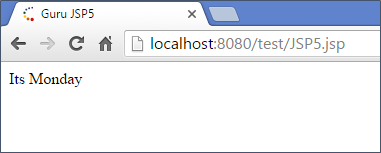• The output of this code will be “its Monday”.
• JSP Operators
• JSP Operators supports most of its arithmetic and logical operators which are supported by java within expression language (EL) tags.
• Frequently used operators are mentioned below:
• Following are the operators:
 . Access a bean property or Map entry [] Access an array or List element ( ) Group a subexpression to change the evaluation order + Addition – Subtraction or negation of a value * Multiplication / or div Division % or mod Modulo (remainder) == or eq Test for equality != or ne Test for inequality < or lt Test for less than > or gt Test for greater than <= or le Test for less than or equal >= or ge Test for greater than or equal && or and Test for logical AND || or or Test for logical OR ! or not Unary Boolean complement Empty Test for empty variable values
• In this example,
• We are declaring two variables num1 and num2 and then take a variable num3, where we use JSP operator + by to add num1 and num2 and get num3.
• Then we check a condition whether num3 is not equal to 0 by using JSP operators (!= , >) and
• Then take another variable num4 by multiplying two num1 and num2 we get num4.
• These all numbers should be printed out as our output:
• <%@ page language=“java” contentType=“text/html; charset=ISO-8859-1”
• pageEncoding=“ISO-8859-1”%>
• <!DOCTYPE html PUBLIC “-//W3C//DTD HTML 4.01 Transitional//EN” “http://www.w3.org/TR/html4/loose.dtd”>
• <html>
• <meta http-equiv=“Content-Type” content=“text/html; charset=ISO-8859-1”>
• <title>Guru JSP6</title>
•
•
• <body>
•
• <% int num1=10; int num2 = 50;
•
• int num3 = num1+num2;
•
• if(num3 != 0 || num3 > 0){
•
• int num4= num1*num2;
•
• out.println(“Number 4 is “ +num4);
• out.println(“Number 3 is “ +num3);
•
• }%>
•
• </body>
•
• </html>
• Explanation of the code:
• Code Line 10: Variable named num1 is initialized to 10 and num2 to 50 in the expression tags
• Code Line 11: The variable num3 is sum of of num1 and num2 where we are using addition operator
• Code Line 12-16: In the EL tags, we have used an OR condition which is logical operator and arithmetic operators to check whether num3 is greater than 0 in the if condition. The OR is used when any one condition is true in such case it will enter the “if case”where we are multiplying two numbers “num1” and “num2” and getting output in “num4″and it will print the output stream.
• When you execute the above code, you will have the following Output.
• Output:
•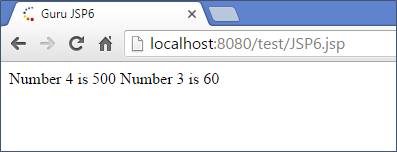• The first output is Number 4 is 500 (variable num4 which is num1*num2)
• The second output is Number 3 is 60 (variable num3 which num1+num2)
• Summary:
• JSP Expression Language (EL) makes it easy to access the application for the data stored in the javabeans components.
• It also allows create the expressions which are both arithmetic and logical.
• Within EL tags we can use integers. Floating point numbers, strings and Boolean values.
• In JSP we can also use loops and decision making statements using EL tags

#### Top Courses